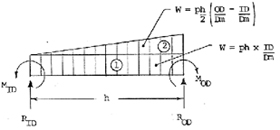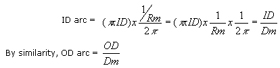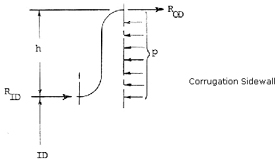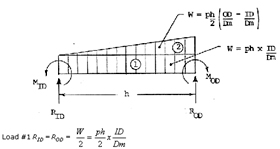Request A Quote

Bellows Expansion Joint: Pressure Loads, Anchors & Guides

AppendixConsidering the pie-shaped strip beam cut out of the corrugation to be a combination of a beam of uniform width and a triangular beam, the reactions “RID” and “ROD” can be determined by superposition*.dSummary: Above bellows thrust load was compared to the mean diameter approximation on a number of sample problems as shown below.Note: For bellows “Effective Area, Ae”, delete “p” from the above equations.

* This solution for reactions assume the cross section varies in such a way that moment of inertia is constant. In a formed bellows, increased circumference is associated with material thinning in such a manner that “I” of crest is approximately equal to “I” of root.

Top